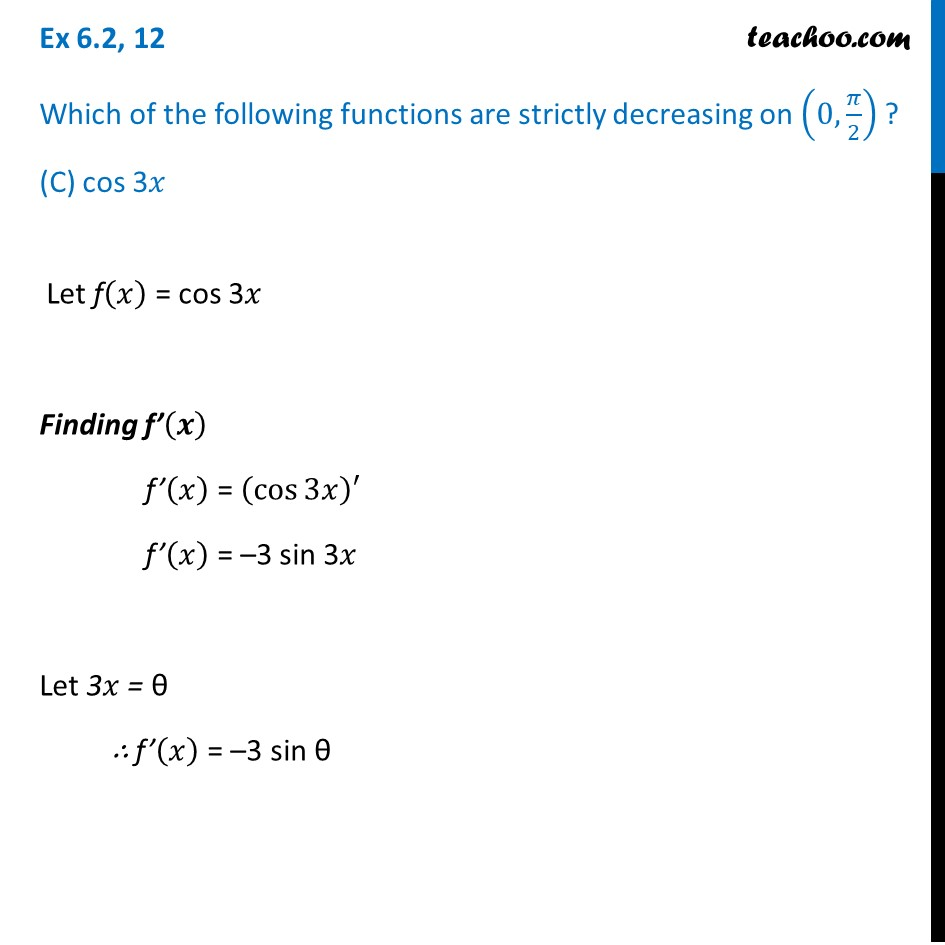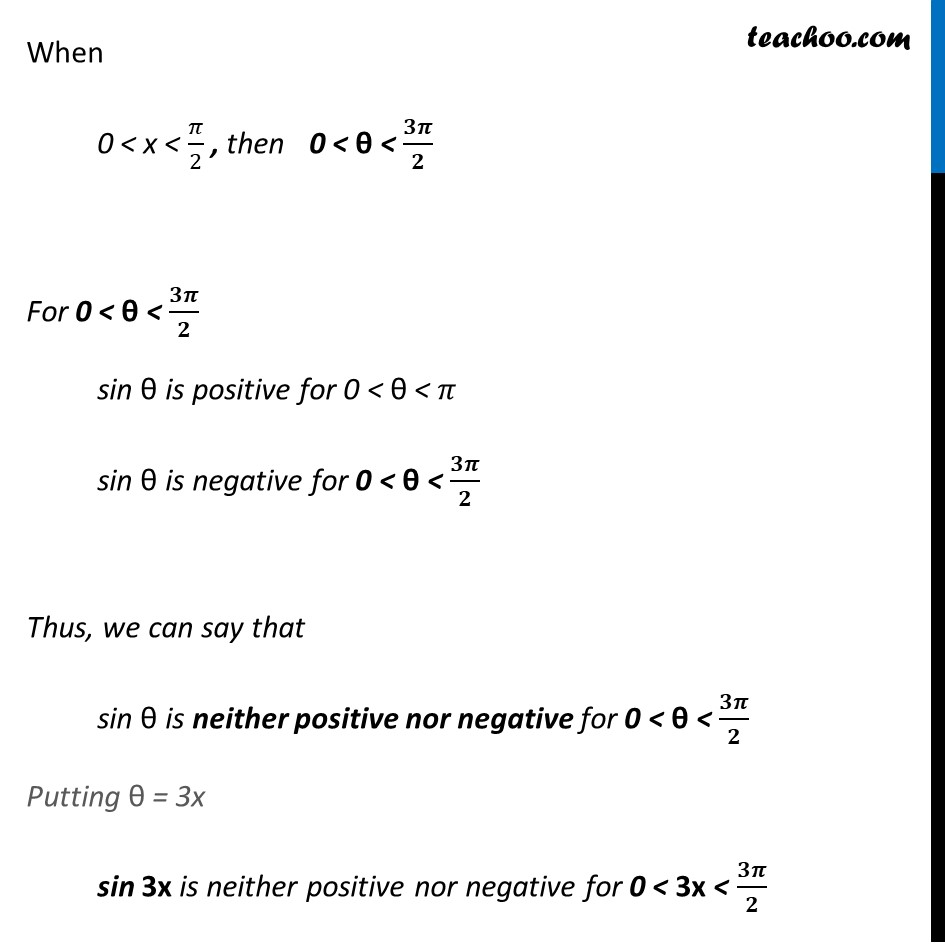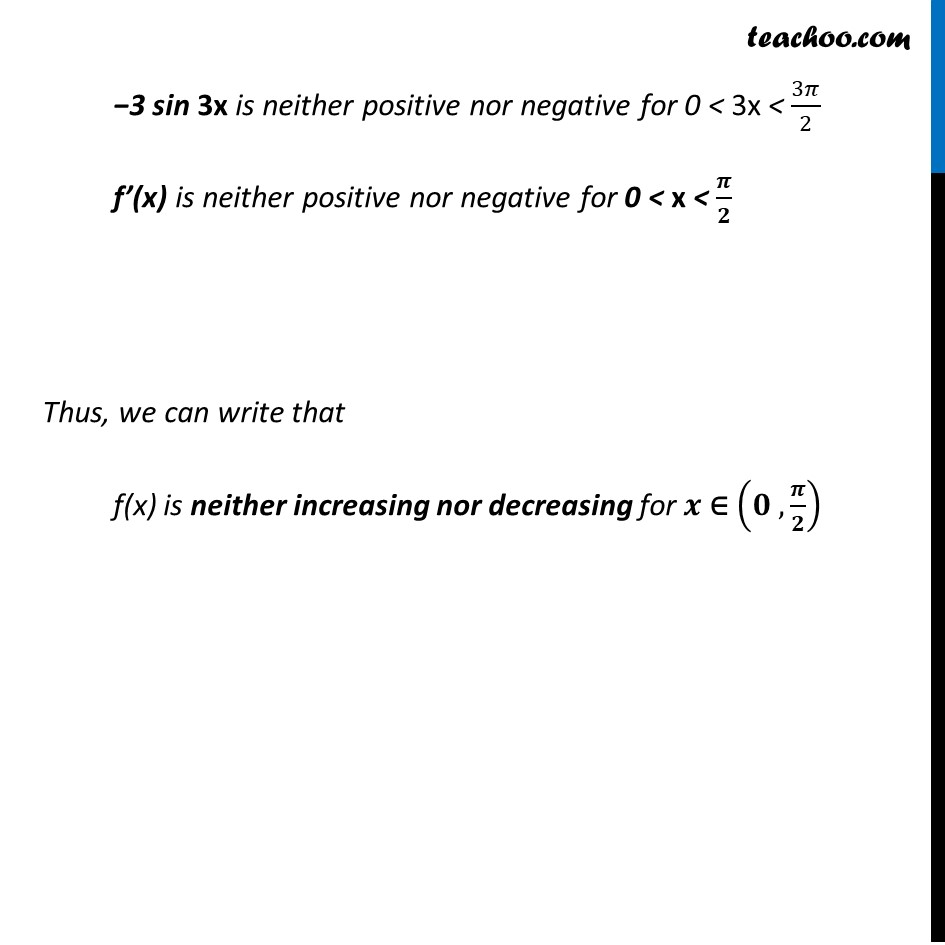Ex 6.2

Chapter 6 Class 12 Application of Derivatives
Serial order wiseGet live Maths 1-on-1 Classs - Class 6 to 12

### Transcript

Ex 6.2, 12 Which of the following functions are strictly decreasing on (0,𝜋/2) ? (C) cos 3𝑥 Let f(𝑥) = cos 3𝑥 Finding f’(𝒙) f’(𝑥) = (cos⁡3𝑥 )′ f’(𝑥) = –3 sin 3𝑥 Let 3𝑥 = θ ∴ f’(𝑥) = –3 sin θ When 0 < x < 𝜋/2 , then 0 < θ < 𝟑𝝅/𝟐 For 0 < θ < 𝟑𝝅/𝟐 sin θ is positive for 0 < θ < 𝜋 sin θ is negative for 0 < θ < 𝟑𝝅/𝟐 Thus, we can say that sin θ is neither positive nor negative for 0 < θ < 𝟑𝝅/𝟐 Putting θ = 3x sin 3x is neither positive nor negative for 0 < 3x < 𝟑𝝅/𝟐 −3 sin 3x is neither positive nor negative for 0 < 3x < 3𝜋/2 f’(x) is neither positive nor negative for 0 < x < 𝝅/𝟐 Thus, we can write that f(x) is neither increasing nor decreasing for 𝒙 ∈ (𝟎 , 𝝅/𝟐)